# Matrix TeacherPage 1

#### WATCH ALL SLIDES

Slide 1Matrix Operations

Slide 2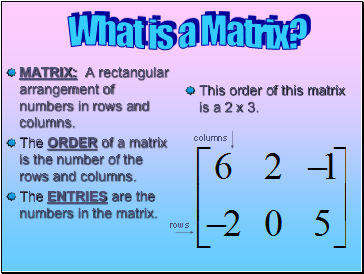## What is a Matrix?

MATRIX: A rectangular arrangement of numbers in rows and columns.

The ORDER of a matrix is the number of the rows and columns.

The ENTRIES are the numbers in the matrix.

rows

columns

This order of this matrix is a 2 x 3.

Slide 3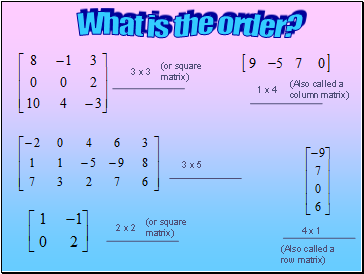## What is the order?

3 x 3

3 x 5

2 x 2

4 x 1

1 x 4

(or square matrix)

(Also called a row matrix)

(or square matrix)

(Also called a column matrix)

Slide 4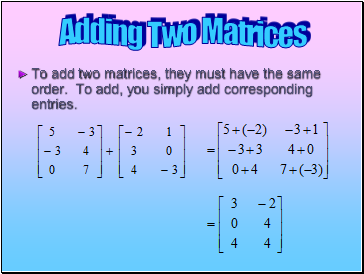To add two matrices, they must have the same order. To add, you simply add corresponding entries.

Slide 5=

=

7

7

4

5

0

7

5

7

Slide 6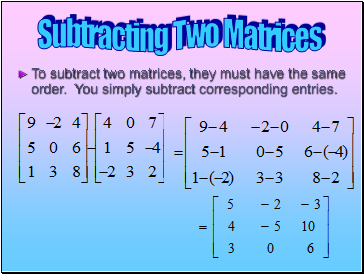## Subtracting Two Matrices

To subtract two matrices, they must have the same order. You simply subtract corresponding entries.

Slide 7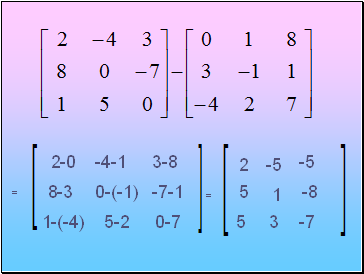=

5-2

-4-1

3-8

8-3

0-(-1)

-7-1

1-(-4)

2-0

0-7

=

2

-5

-5

5

1

-8

5

3

-7

Slide 8## Multiplying a Matrix by a Scalar

In matrix algebra, a real number is often called a SCALAR. To multiply a matrix by a scalar, you multiply each entry in the matrix by that scalar.

Slide 9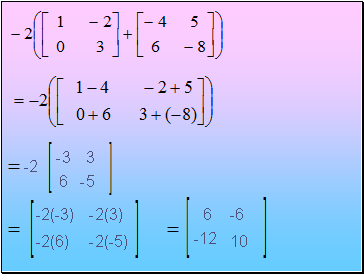-2

6

-3

3

-2(-3)

-5

-2(6)

-2(-5)

-2(3)

6

-6

-12

10